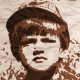# Extract point cloud points from the output matrix of Linear Triangulation methodI'm trying to reconstruct 3D object in XNA. I need point cloud point for that. I implemented the concept under uncalibrated image sequence for 3d reconstruction. I'm stuck with Linear Triangulation now. As a result I have value with matrix. What is my next step to get list of (x,y,z) points to draw the mesh. It will be really great full if some one help me. Struggling in this stage for more than one week.

edit retag close merge delete

Can you please more specific? What is the matrix you refer to? Is it a fundamental matrix for 2 images?

My Reconstruction Pipe Line for uncaliberared images as follows 1) Matched the features using SURF and got the corresponding points. 2) Using the corresponding points compute the fundamental matrix. 3) Calculate the camera matrix from fundamental matrix (Return camera matrix based on "Multiple View Geometry" by Hartley and Zisserman) 4) Then Linear Triangulation, Opencv has triangulatePoints method. I used this method to trangulate the points. It’s returning a Matrix value.

So far this what I have implemented. Is it correct? What is my next step? I'm stuck in trangulate point matrix. How can I get the vertex points? So i can pass it to XNA and draw the mesh.

Sort by » oldest newest most votedMaybe I'm missing something but it's not possible to perform metric reconstruction from 2 uncalibrated images (unless you make some additional assumptions, e.g. you know/estimate location of a vanishing point or you known something about camera calibration). You can make reconstruction up to a projective transformation. See projective reconstruction theorem in Harley book.

How do you calculate camera matrices from fundamental matrix? What formula are you using? Without knowing camera intrinsic matrix it's not possible. Are you really sure this step is correct?

triangulatePoints returns homogeneous coordinates of 3D points, one point in a column.

Homogeneous coordinate(X,Y,Z,W) = euclidean coordinate (X/W, Y/W, Z/W).

more

Thanks for your detail answer I will recheck on it. Ya you’re correct. I’m still in the starting level. I have planned to use un calibrated image sequence ( 50 images). Yes I looked in to projective reconstruction with 2 images. If I’m not wrong - Returns two camera matrices based on result 9.14 from "Multiple View Geometry" by Hartley and Zisserman (P = [I | 0] and P' = [[e']×F | e']). This concept comes under, I think structure from motion. Oh ok. I understand this point. Any idea of self-camera calibrations of the image?? To get a robust 3D model. Most of the research papers recommend this. Any idea of implementing it???

Official site

GitHub

Wiki

Documentation